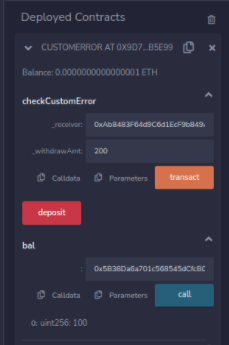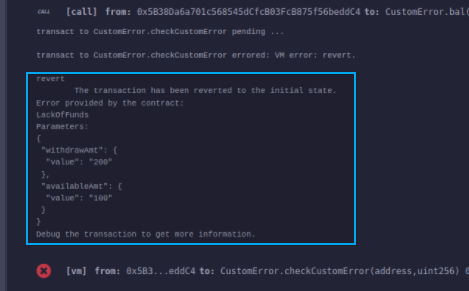Related Articles

# Solidity – Error Handling

Solidity has many functions for error handling. Errors can occur at compile time or runtime. Solidity is compiled to byte code and there a syntax error check happens at compile-time, while runtime errors are difficult to catch and occurs mainly while executing the contracts. Some of the runtime errors are out-of-gas error, data type overflow error, divide by zero error, array-out-of-index error, etc. Until version 4.10 a single throw statement was there in solidity to handle errors, so to handle errors multiple if…else statements, one has to implement for checking the values and throw errors which consume more gas. After version 4.10 new error handling construct assert, require, revert statements were introduced and the throw was made absolute.

### Require Statements

The ‘require’ statements declare prerequisites for running the function i.e. it declares the constraints which should be satisfied before executing the code. It accepts a single argument and returns a boolean value after evaluation, it also has a custom string message option. If false then exception is raised and execution is terminated. The unused gas is returned back to the caller and the state is reversed to its original state. Following are some cases when require type of exception is triggered :

• When require() is called with the arguments which result as false.
• When a function called by a message does not end properly.
• When a contract is created using the new keyword and the process does not end properly.
• When a codeless contract is targeted to an external function.
• When ethers are sent to the contract using the public getter method.
• When .transfer() method fails.
• When an assert is called with a condition that results in false.
• When a zero-initialized variable of a function is called.
• When a large or a negative value is converted to an enum.
• When a value is divided or modulo by zero.
• When accessing an array in an index which is too big or negative.

Example: In the below example, the contract requireStatement demonstrates how to use the ‘require statement’.

## Solidity

 `// Solidity program to demonstrate require statement` `pragma solidity ^0.5.0;`   `// Creating a contract` `contract` `requireStatement {` `   `  `    ``// Defining function to check input ` `    ``function checkInput(uint _input) ``public` `view returns(string memory){` `        ``require(_input >= 0, ``"invalid uint8"``);` `        ``require(_input <= 255, ``"invalid uint8"``);` `        `  `        ``return` `"Input is Uint8"``;` `    ``}` `   `  `    ``// Defining function to use require statement` `    ``function Odd(uint _input) ``public` `view returns(``bool``){` `        ``require(_input % 2 != 0);` `        ``return` `true``;` `    ``}` `}`

Output :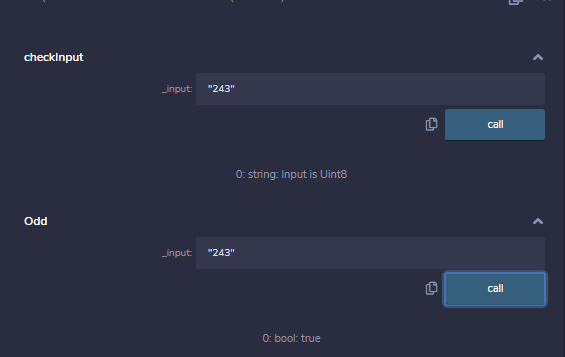### Assert Statement

Its syntax is similar to the require statement. It returns a boolean value after the evaluation of the condition. Based on the return value either the program will continue its execution or it will throw an exception. Instead of returning the unused gas, the assert statement consumes the entire gas supply and the state is then reversed to the original state. Assert is used to check the current state and function conditions before the execution of the contract. Below are some cases with assert type exceptions :

• When an assert is called with a condition that results in false.
• When a zero-initialized variable of a function is called.
• When a large or a negative value is converted to an enum.
• When a value is divided or modulo by zero.
• When accessing an array in an index which is too big or negative.

Example: In the below example, the contract assert Statement demonstrates how to use an ‘assert statement’.

## Solidity

 `// Solidity program to demonstrate assert statement ` `pragma solidity ^0.5.0;`   `// Creating a contract` `contract` `assertStatement {` `  `  `    ``// Defining a state variable  ` `    ``bool` `result;`   `    ``// Defining a function to check condition` `    ``function checkOverflow(uint _num1, uint _num2) ``public` `{` `        ``uint sum = _num1 + _num2;` `        ``assert``(sum<=255);` `        ``result = ``true``;` `    ``}` `   `  `    ``// Defining a function to print result of assert statement` `    ``function getResult() ``public` `view returns(string memory){` `         ``if``(result == ``true``){` `             ``return` `"No Overflow"``;` `         ``}` `         ``else``{` `             ``return` `"Overflow exist"``;` `         ``}` `    ``}` `}`

Output :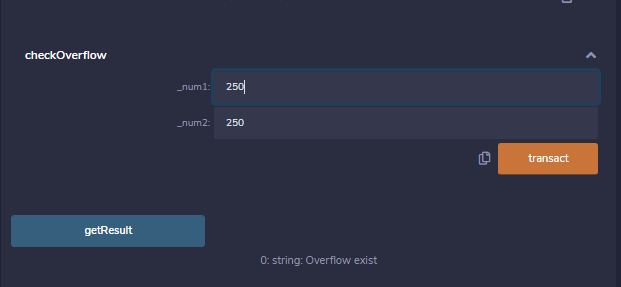### Revert Statement

This statement is similar to the require statement. It does not evaluate any condition and does not depends on any state or statement. It is used to generate exceptions, display errors, and revert the function call. This statement contains a string message which indicates the issue related to the information of the exception. Calling a revert statement implies an exception is thrown, the unused gas is returned and the state reverts to its original state.  Revert is used to handle the same exception types as require handles, but with little bit more complex logic.

Example: In the below example, the contract revertStatement demonstrates the ‘revert statement’.

## Solidity

 `// Solidity program to demonstrate revert statement` `pragma solidity ^0.5.0;`   `// Creating a contract` `contract` `revertStatement {` `  `  `   ``// Defining a function to check condition ` `   ``function checkOverflow(uint _num1, uint _num2) ``public` `view returns(string memory, uint){` `        ``uint sum = _num1 + _num2;` `         ``if``(sum < 0 || sum > 255){` `             ``revert(``" Overflow Exist"``);` `         ``}` `         ``else``{` `             ``return` `(``"No Overflow"``, sum);` `         ``}` `         `  `    ``}` `    `  `}` `    `  `}`

Output :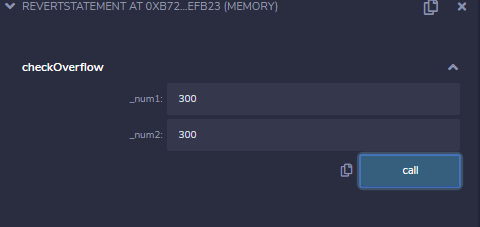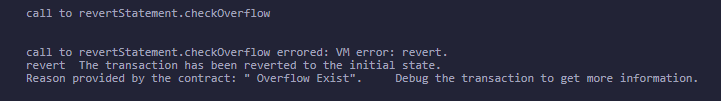### Custom Error

To define the custom error in the smart contract by using the “Error statement” . Error statement is used either inside the smart contract or outside the smart contract along with revert statement. The main purpose of using custom error in the smart contract is to save gas.

Example : In the below example some amount is deposited to the owner address and if owner wants to transfer this amount to given address than first we checks withdraw amount is less than owner address amount if the condition is matched than withdraw amount is successfully transferred to the given address. Otherwise, If the condition is not matched than transaction is reverted with the help of Error statement provided by the contract.

## Solidity

 `// SPDX-License-Identifier: GPL-3.0 ` `pragma solidity >=0.4.22 <0.9.0;  ` `/// @title A contract for demonstrate custom error ` `/// @author Jitendra Kumar` `/// @notice For now, this contract just show how custom error generates if owner address amount is less than withdraw amount ` `contract` `CustomError {` `    ``//mapping` `    ``mapping(address => uint) ``public` `bal;`   `    ``//amount deposit to owner address` `    ``function deposit() ``public` `payable {` `        ``bal[msg.sender] += msg.value;` `    ``} `   `    ``// custom error statement` `    ``error LackOfFunds(uint withdrawAmt, uint availableAmt);` `    `  `    ``//custom error generates if withdraw amount is greater than owner address amount` `    ``function checkCustomError(address _receiver,uint _withdrawAmt) ``public` `{` `        ``if` `(bal[msg.sender]< _withdrawAmt) {` `            ``revert LackOfFunds({withdrawAmt: _withdrawAmt, availableAmt: bal[msg.sender]});` `        ``}` `        ``bal[msg.sender] -= _withdrawAmt;` `        ``bal[_receiver]+=_withdrawAmt;` `    ``}` `}`

Output:

transact to CustomError.checkCustomError errored: VM error: revert.

revert
The transaction has been reverted to the initial state.
Error provided by the contract:
LackOfFunds
Parameters:
{
“withdrawAmt”: {
“value”: “200”
},
“availableAmt”: {
“value”: “100”
}
}